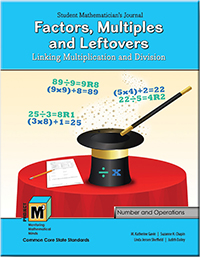# Project M³ Curriculum Units — Level 3-4

## Factors, Multiples and Leftovers: Linking Multiplication and DivisionIn this unit, students develop their number sense with a focus on a deeper understanding of multiplication and division. They encounter a range of different problem situations and representations and learn about the relationship between multiplication and division and the properties associated with these operations. Rather than focusing on multiplication facts, Factors, Multiples and Leftovers: Linking Multiplication and Division focuses on the development of students’ multiplicative reasoning power. This goes far beyond computation to a solid understanding of the meaning of the operation and an ability to recognize problem situations where multiplication is appropriate. Fluency with multiplication is stressed through constructing and analyzing area models of multiplication. Using such models, properties of multiplication such as the commutative property become obvious. Other relationships such as the distributive property of multiplication over addition can be seen by composing and decomposing area models. With our emphasis on providing challenges to students with talent, we extend student thinking about multiplication to factors and multiples and also look at relationships among prime, composite, square, odd and even numbers. In the final lesson of the chapter, they write their own training manual, Strategies for Becoming a Multiplying Magician, to highlight their new facility with and understanding of multiplication.

Multiplication is connected to division in Chapter 2. Explored as the inverse of multiplication, division is again presented in the context of developing number sense. Students learn how to work with ‘near facts’ such as 50 ÷ 8 which is near 48 ÷ 8. This is an initiation into the study of remainders, which is a theme throughout the second chapter. Again, the emphasis is always on the meaning of division and making sense of a situation. The role of the remainder is very important in helping students interpret the solution to problem situations that require division. Sometimes the remainder should be recorded as a fraction, sometimes as a decimal, sometimes it should be used to round the quotient to a whole number, and sometimes the remainder is actually the solution. Students need to evaluate each situation to see what the answer to the problem requires. For example, if the problem discusses how many cars are needed to transport a group of students to an event, the answer must be a whole number and be greater than the quotient. We cannot have 1⁄2 of a car!